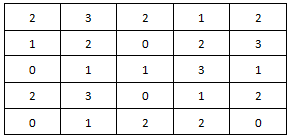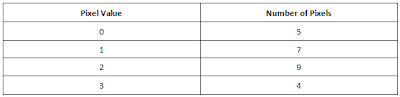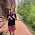### Histogram Equalization

Histogram of an image is the graphical representation of the distribution of intensities of pixels. It provides an estimate of where pixel values are concentrated and whether there are unusual deviations.

e.g. - Consider the following image. Say, all pixel values have a depth of 2 bits and are unsigned. Therefore the allowable value range of pixels is  0 - 3.Sample Image

As you can see in the above image, there are 5 pixels with value 0, 7 pixels with value 1, 9 pixels with value 2 and 4 pixels with value 3. These information is tabulated as follows.Intensity distribution of the above image

The histogram of an image is usually presented as a graph. The following graph represents the histogram of the above image.Image Histogram

Histogram equalization is a commonly used technique in image processing to enhance the contrast of an image by equalizing the intensity distribution. It will make a dark image (underexposed) less dark and a bright image (overexposed) less bright.

The equalized histogram of the above image should be ideally like the following graph.Ideal histogram of the image after the equalization

But practically, it is hard to achieve this kind of perfect histogram equalization. However there are various techniques to achieve histogram equalization close to the perfect one. In OpenCV, there is an in-built function to equalize the histogram.

### Histogram Equalization of a Grayscale image with OpenCV

Here is the example program demonstrating how to equalize the histogram of a grayscale image using the OpenCV in-built function.

```//Uncomment the following line if you are compiling this code in Visual Studio
//#include "stdafx.h"

#include <opencv2/opencv.hpp>
#include <iostream>

using namespace cv;
using namespace std;

int main(int argc, char** argv)
{
// Read the image file
Mat image = imread("D:/My OpenCV Website/fly-agaric.jpg");

// Check for failure
if (image.empty())
{
cout << "Could not open or find the image" << endl;
cin.get(); //wait for any key press
return -1;
}

//change the color image to grayscale image
cvtColor(image, image, COLOR_BGR2GRAY);

//equalize the histogram
Mat hist_equalized_image;
equalizeHist(image, hist_equalized_image);

//Define names of windows
String windowNameOfOriginalImage = "Original Image";
String windowNameOfHistogramEqualized = "Histogram Equalized Image";

// Create windows with the above names
namedWindow(windowNameOfOriginalImage, WINDOW_NORMAL);
namedWindow(windowNameOfHistogramEqualized, WINDOW_NORMAL);

// Show images inside created windows.
imshow(windowNameOfOriginalImage, image);
imshow(windowNameOfHistogramEqualized, hist_equalized_image);

waitKey(0); // Wait for any keystroke in one of the windows

destroyAllWindows(); //Destroy all open windows

return 0;
}
```
Copy and paste the above code snippet into your IDE and run it. Please note that you have to replace "D:/My OpenCV Website/fly-agaric.jpg" in the code with a valid location to an image in your computer. Then you should see 2 images like the below.Histogram Equalization of Grayscale Image

#### Explanation

Let's go through the above code line by line.

```// Read the image file
Mat image = imread("D:/My OpenCV Website/fly-agaric.jpg");

// Check for failure
if (image.empty())
{
cout << "Could not open or find the image" << endl;
cin.get(); //wait for any key press
return -1;
}
```

The above code segment will load the image from the specified file. The program will exit if the image load-up is failed.

```//change the color image to grayscale image
cvtColor(image, image, COLOR_BGR2GRAY);
```
The above function converts the image in BGR color space to grayscale color space.

Please note that the color space of the loaded image is BGR, not RGB. (i.e. - Channels are ordered as blue, green and red.)

```//equalize the histogram
Mat hist_equalized_image;
equalizeHist(image, hist_equalized_image);
```
The above function equalizes the histogram of the grayscale image and store the output in the hist_equalized_image.

```//Define names of windows
String windowNameOfOriginalImage = "Original Image";
String windowNameOfHistogramEqualized = "Histogram Equalized Image";

// Create windows with the above names
namedWindow(windowNameOfOriginalImage, WINDOW_NORMAL);
namedWindow(windowNameOfHistogramEqualized, WINDOW_NORMAL);

// Show images inside the created windows.
imshow(windowNameOfOriginalImage, image);
imshow(windowNameOfHistogramEqualized, hist_equalized_image);
```
The above code segment will create windows and show images in them. As windows are created passing the flag WINDOW_NORMAL, they can be resized freely.

```waitKey(0); // Wait for any keystroke in the window

destroyAllWindows(); //destroy all open windows

return 0;
```
The program will wait until any key is pressed. After a key is pressed, all created windows will be destroyed and the program will exit.

#### Summary

In the above example, you have learnt how to
• Load an image from the file
• Convert a color image to a grayscale image
• Equalize the histogram of a grayscale image
• Create windows and show images in them
• Keep your program waiting for a key press
• Destroy all created windows

### Histogram Equalization of a Color image with OpenCV

In this example, I will show you how to equalize the histogram of a color image using simple OpenCV program.

```//Uncomment the following line if you are compiling this code in Visual Studio
//#include "stdafx.h"

#include <opencv2/opencv.hpp>
#include <iostream>

using namespace cv;
using namespace std;

int main(int argc, char** argv)
{
// Read the image file
Mat image = imread("D:/My OpenCV Website/fly-agaric.jpg");

// Check for failure
if (image.empty())
{
cout << "Could not open or find the image" << endl;
cin.get(); //wait for any key press
return -1;
}

//Convert the image from BGR to YCrCb color space
Mat hist_equalized_image;
cvtColor(image, hist_equalized_image, COLOR_BGR2YCrCb);

//Split the image into 3 channels; Y, Cr and Cb channels respectively and store it in a std::vector
vector<Mat> vec_channels;
split(hist_equalized_image, vec_channels);

//Equalize the histogram of only the Y channel
equalizeHist(vec_channels, vec_channels);

//Merge 3 channels in the vector to form the color image in YCrCB color space.
merge(vec_channels, hist_equalized_image);

//Convert the histogram equalized image from YCrCb to BGR color space again
cvtColor(hist_equalized_image, hist_equalized_image, COLOR_YCrCb2BGR);

//Define the names of windows
String windowNameOfOriginalImage = "Original Image";
String windowNameOfHistogramEqualized = "Histogram Equalized Color Image";

// Create windows with the above names
namedWindow(windowNameOfOriginalImage, WINDOW_NORMAL);
namedWindow(windowNameOfHistogramEqualized, WINDOW_NORMAL);

// Show images inside the created windows.
imshow(windowNameOfOriginalImage, image);
imshow(windowNameOfHistogramEqualized, hist_equalized_image);

waitKey(0); // Wait for any keystroke in any one of the windows

destroyAllWindows(); //Destroy all opened windows

return 0;
}
```
Copy and paste the above code snippet into your IDE and run it. Please note that you have to replace "D:/My OpenCV Website/fly-agaric.jpg" in the code with a valid location to an image in your computer. Then you should see 2 images like the below.Histogram equalization of a color image

#### Explanation

OpenCV loads color images in BGR color space. With this color space, it is not possible to equalize the histogram without affecting to the color information because all 3 channels contain color information. Therefore you have to convert the BGR image to a color space like YCrCb. In YCrCb color space, the Y channel of the image only contains intensity information where as Cr and Cb channels contain all the color information of the image. Therefore only the Y channel should be processed to get a histogram equalized image without changing any color information. After the processing, the YCrCb image should be converted back to the BGR color space before calling imshow() function.

Let's go through the code line by line.

```// Read the image file
Mat image = imread("D:/My OpenCV Website/fly-agaric.jpg");

// Check for failure
if (image.empty())
{
cout << "Could not open or find the image" << endl;
cin.get(); //Wait for any key press
return -1;
}
```
The above code segment loads the image from the specified file. If it is failed to load the image, the program will exit.

```//Convert the image from BGR to YCrCb color space
Mat hist_equalized_image;
cvtColor(image, hist_equalized_image, COLOR_BGR2YCrCb);
```
The loaded image is in BGR color space. None of the 3 channels (blue, green and red) of this color space cannot be processed to equalize the histogram because all the channels contain color information. Therefore the loaded image should be converted to the YCrCb color space. In this color space, Y channel only contains intensity information while Cr and Cb channels contain color information. Therefore only the Y channel needs to be processed in order to equalize the histogram.

```//Split the image into 3 channels; Y, Cr and Cb channels respectively and store it in a std::vector
vector<Mat> vec_channels;
split(hist_equalized_image, vec_channels);
```
The above OpenCV function splits the 3 channel image into 3 separate matrices. Each matrix is pushed to the std::vector. vec_channels contains the Y channel, vec_channels contains the Cr channel and vec_channels contains the Cb channel.

```//Equalize the histogram of the Y channel
equalizeHist(vec_channels, vec_channels);
```
The above function equalizes the histogram of the Y channel.

```//Merge 3 channels in the vector to form the color image in YCrCB color space.
merge(vec_channels, hist_equalized_image);
```
The above function performs the reverse operation of the split function. It takes a std::vector which consists of 3 matrices representing Y, Cr and Cb channels and creates a 3 channel image in YCrCb color space.

```//Convert the histogram equalized image from YCrCb to BGR color space again
cvtColor(hist_equalized_image, hist_equalized_image, COLOR_YCrCb2BGR);
```
Above line converts the image in YCrCb color space into the BGR color space. This step is necessary because OpenCV functions like cv::imshow() always expect images in BGR color space.

```//Define the names of windows
String windowNameOfOriginalImage = "Original Image";
String windowNameOfHistogramEqualized = "Histogram Equalized Color Image";

// Create windows with the above names
namedWindow(windowNameOfOriginalImage, WINDOW_NORMAL);
namedWindow(windowNameOfHistogramEqualized, WINDOW_NORMAL);

// Show images inside the created windows
imshow(windowNameOfOriginalImage, image);
imshow(windowNameOfHistogramEqualized, hist_equalized_image);
```
The above code segment will create windows and show images in them. As both windows are created passing the flag WINDOW_NORMAL, they can be resized freely.

```waitKey(0); // Wait for any keystroke in any one of the windows

destroyAllWindows(); //Destroy all opened windows

return 0;
```
The program will wait until any key is pressed. After a key is pressed, all created windows will be destroyed and the program will exit.

#### Summary

In the above example, you have learnt how to
• Load an image from a file
• Identify a color space suitable for histogram equalization
• Change color space of an image
• Split a multi-channel image into separate matrices
• Equalize the histogram of a specific channel without affecting to other channels
• Merge separate matrices into a multi-channel image
• Create windows and show images in them
• Keep your program waiting for a key press
• Destroy all created windows

### Histogram Equalization of a Video with OpenCV

Now I am going to show you how to equalize the histogram of a video using an OpenCV C++ example. This is pretty much similar to the previous example.

It is recommended to go through the Play Video from File or Camera first in order to understand the following example better.

```//Uncomment the following line if you are compiling this code in Visual Studio
//#include "stdafx.h"

#include <opencv2/opencv.hpp>
#include <iostream>

using namespace cv;
using namespace std;

int main(int argc, char* argv[])
{
//Open the video file for reading
VideoCapture cap("D:/My OpenCV Website/A Herd of Deer Running.mp4");

// if not success, exit the program
if (cap.isOpened() == false)
{
cout << "Cannot open the video file" << endl;
cin.get(); //wait for any key press
return -1;
}

//Define the names of windows
String windowNameOfOriginalImage = "Original Video";
String windowNameOfHistogramEqualized = "Histogram Equalized Video";

// Create windows with the above names
namedWindow(windowNameOfOriginalImage, WINDOW_NORMAL);
namedWindow(windowNameOfHistogramEqualized, WINDOW_NORMAL);

while (true)
{
Mat frame;
bool bSuccess = cap.read(frame); // Read a new frame from the video file

//Breaking the while loop at the end of the video
if (bSuccess == false)
{
cout << "Found the end of the video" << endl;
break;
}

//Convert the frame from BGR to YCrCb color space
Mat hist_equalized_image;
cvtColor(frame, hist_equalized_image, COLOR_BGR2YCrCb);

//Split the image into 3 channels; Y, Cr and Cb channels respectively and store it in a std::vector
vector<Mat> vec_channels;
split(hist_equalized_image, vec_channels);

//Equalize the histogram of the Y channel
equalizeHist(vec_channels, vec_channels);

//Merge 3 channels in the std::vector to form the color image in YCrCB color space.
merge(vec_channels, hist_equalized_image);

//Convert the histogram equalized image from YCrCb to BGR color space again
cvtColor(hist_equalized_image, hist_equalized_image, COLOR_YCrCb2BGR);

//show frames in the created windows
imshow(windowNameOfOriginalImage, frame);
imshow(windowNameOfHistogramEqualized, hist_equalized_image);

//Wait for 10 ms until any key is pressed.
//If the 'Esc' key is pressed, break the while loop.
//If the any other key is pressed, continue the loop
//If any key is not pressed within 10 ms, continue the loop
if (waitKey(5) == 27)
{
cout << "Esc key is pressed by the user. Stopping the video" << endl;
break;
}
}

destroyAllWindows(); //Destroy all opened windows

return 0;

}
```

Copy and paste the above code snippet into your IDE and run it. Please note that you have to replace "D:/My OpenCV Website/A Herd of Deer Running.mp4" in the code with a valid location to a video in your computer. Then you should see two videos, the histogram equalized video and the original video.

Next Lesson: Filter Images and Videos

#### 4 comments:

1.during executing "Histogram Equalization of a Color image with OpenCV", i am getting this error "Windows has triggered a breakpoint in HelloWorld.exe................... and the program is not showing output

2.I found useful article in your blog. thank you for sharing nice info
visit
web programming tutorial
welookups.com

3.How to perform the histogram equalization on each of the three components separately for color image？

1.I can't say how to do that with OpenCV, but you wouldn't want to as it will distort the colors in your image and make it unrecognizable. The goal of histogram equalization is to increase contrast between features which is done in a single dimension.